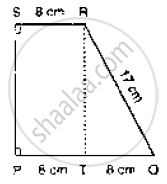# Compute the Area of Trapezium Pqrs is Fig. Below. - Mathematics

Compute the area of trapezium PQRS is Fig. below.#### Solution

We have

ar (trap PQRS)  =  ar  (rect PSRT) +  are  a  (ΔQRT)

⇒ ar   (trap  .PQRS)  = PT × RT =1/2(QT × RT)

=   8 xx RT = 1/2 ( 8 xx RT ) = 12 xx RT

In , ΔQRT , we have

QR2 = QT2 + RT2

⇒ RT = QR2  - QT2

⇒ ( RT ) = 172 - 8 2 = 225

⇒ RT = 15

Hence, ar (trap . PQRS ) = 12 × 15cm = 180 cm2

Concept: Concept of Area
Is there an error in this question or solution?
Chapter 14: Areas of Parallelograms and Triangles - Exercise 14.3 [Page 44]

#### APPEARS IN

RD Sharma Mathematics for Class 9
Chapter 14 Areas of Parallelograms and Triangles
Exercise 14.3 | Q 3 | Page 44
Share